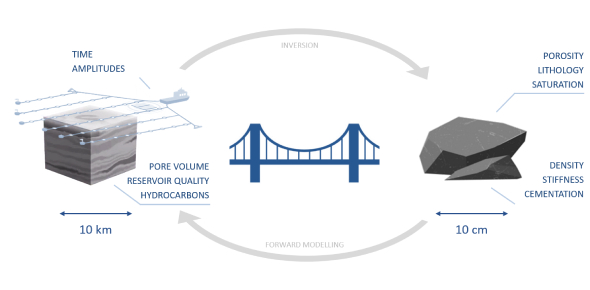# Rock Physics Trivia: MCQ Quiz!

21 Questions | Total Attempts: 174SettingsCreate your own Quiz.

• 1.
Calculate the Acoustic Impedance of sand interval with velocity 2500 m/s and density 2.0 g/cc.
• A.

2500 m/s gr/cc

• B.

5000 m/s kg/m3

• C.

5 x 10^6 m/s kg/m3

• D.

2500 m/s kg/m3

• 2.
What is the preference wavelet phase for Seismic Interpretation?
• A.

Maximum Phase

• B.

American convension

• C.

Zero Phase

• D.

Minimum Phase

• 3.
Rock Physics is a branch of science for:
• A.

Understanding the seismic survey

• B.

Understanding the relationship between geophysical measurement and rock properties

• C.

Understanding the relationship between geophysical measurement and the location

• D.

Maximizing the risk in hydrocarbon exploration

• 4.
What is the main difference between Petrophysics and Rock Physics?
• A.

Petrophysics more emphasis on stress, faulting, fracture processes

• B.

Petrophysics more emphasis on interpreting seismic, sonic and ultrasonic data

• C.

Petrophysics more emphasis on well logs, and often ignoring sonic logs and seismic

• D.

Petrophysics more emphasis on seismic survey

• 5.
Petrophysical / Geological Model can be used to differentiate.
• A.

DHI

• B.

AVO Classes

• C.

Depth Trends

• D.

All are correct

• 6.
Which of the following giving negative amplitude response (SEG polarity, Period: Tertiary)?
• A.

Shale overlying Reservoir Gas Sand

• B.

Shale overlying soft shale

• C.

Shale overlying coal

• D.

All are correct

• 7.
The velocity responses to rocks based on, EXCEPT:
• A.

Porosity and pore geometry

• B.

Fluid type and saturation

• C.

Type of acquisition

• D.

Mineral composition and distribution

• 8.
In terms of effective porosity, choose the correct explanation about cementation:
• A.

Loose become stable

• B.

Compaction

• C.

Overburden

• D.

Deposition of dissolved material

• 9.
Reservoir porosity can be classified to several classes, choose the porosity value for very good reservoir.
• A.

0-5 %

• B.

Below 15%

• C.

Above 20%

• D.

Between 5 to 10%

• 10.
Choose the correct answer for the production from unconventional reservoir.
• A.

The hydrocarbon produced from low porosity formation through secondary porosity

• B.

The hydrocarbon produced from low porosity formation through primary porosity

• C.

Brittleness index is not important

• D.

None of the above

• 11.
Choose the correct answer for density definition.
• A.

Ratio between shear and bulk modulus

• B.

Ratio between bulk modulus and poisson ratio

• C.

Ratio between porosity and volume

• D.

Ratio between mass and volume

• 12.
Choose the correct statement about density.
• A.

Density of quartz mineral (zero porosity ) is 2.65 gr/cc

• B.

Density of calcite (zero porosity) is 2.71 gr/cc

• C.

Density is the ratio between mass and volume

• D.

All of the above

• 13.
Choose the correct statement on Bulk Modulus.
• A.

Bulk modulus of water is zero

• B.

Bulk Modulus of gas is higher than oil

• C.

Bulk Modulus of gas is higher than water

• D.

Bulk Modulus of water is higher than gas

• 14.
Poisson Ratio is
• A.

The ratio of extensional stress to extensional strain in a uniaxial stress state

• B.

The ratio of the lateral strain to axial strain in a uniaxial stress state

• C.

The ratio of the pressure applied to the amount of volume change in the cube

• D.

The ratio of shear wave velocity

• 15.
Choose the correct statement about Vp/Vs.
• A.

Can be used to separate different rock type and fluids

• B.

The response can differentiate between coal and gas sand

• C.

The response can be created from slowness logs: shear and primary

• D.

All of the above

• 16.
Young Modulus is
• A.

The ratio of extensional stress to extensional strain in a uniaxial stress state

• B.

The ratio of the lateral strain to axial strain in a uniaxial stress state

• C.

The ratio of the pressure applied to the amount of volume change in the cube

• D.

The ratio of shear wave velocity

• 17.
The Quartz minerals have:
• A.

High Poisson’s Ratio

• B.

Low Poisson’s Ratio

• C.

Higher Poisson’s Ratio than clay minerals

• D.

High Poisson’s Ratio compare to Calcite

• 18.
In shallow high porosity sand region:
• A.

The AI of gas sand lower than shale formation

• B.

The AI of gas sand lower than brine sand

• C.

The Vp/Vs of gas sand lower than brine sand

• D.

All of the above

• 19.
In low porosity sand region:
• A.

The AI of brine sand lower than shale formation

• B.

The AI of gas sand lower than brine sand

• C.

The Vp/Vs of gas sand higher than brine sand

• D.

All of the above

• 20.
Empirical models used in Rock Physics, EXCEPT:
• A.

Castagna’s equation

• B.

Wyllie’s equation

• C.

Han’s equation

• D.

Voigt and Reuss

• 21.
Castagna’s empirical relations:
• A.

Predict the shear velocity from compressional velocity

• B.

Predict porosity from compressional velocity

• C.

Predict clay content from compressional velocity

• D.

Predict Vp from compressional velocity

Related TopicsBack to top
×

Wait!
Here's an interesting quiz for you.